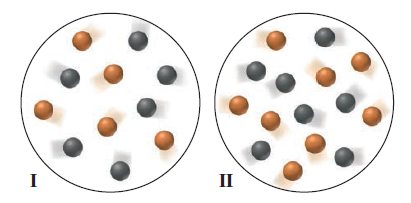# Problem: The following molecular scenes represent starting mixtures I and II for the reaction of A (black) with B (orange):Each sphere represents 0.010 mol, and the volume is 0.50 L. If the reaction is first order in A and first order in B and the initial rate for I is 8.3 x 10-4 mol/L•min, what is the initial rate for II?

###### FREE Expert Solution
94% (373 ratings)
###### Problem Details

The following molecular scenes represent starting mixtures I and II for the reaction of A (black) with B (orange):Each sphere represents 0.010 mol, and the volume is 0.50 L. If the reaction is first order in A and first order in B and the initial rate for I is 8.3 x 10-4 mol/L•min, what is the initial rate for II?

Frequently Asked Questions

What scientific concept do you need to know in order to solve this problem?

Our tutors have indicated that to solve this problem you will need to apply the Rate Law concept. You can view video lessons to learn Rate Law. Or if you need more Rate Law practice, you can also practice Rate Law practice problems.

What textbook is this problem found in?

Our data indicates that this problem or a close variation was asked in Chemistry: The Molecular Nature of Matter and Change - Silberberg 8th Edition. You can also practice Chemistry: The Molecular Nature of Matter and Change - Silberberg 8th Edition practice problems.